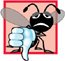# Function Templates

Overloaded functions are normally used to perform similar operations that involve different program logic on different data types. If the program logic and operations are identical for each data type, overloading may be performed more compactly and conveniently by using function templates. The programmer writes a single function template definition. Given the argument types provided in calls to this function, C++ automatically generates separate function template specializations to handle each type of call appropriately. Thus, defining a single function template essentially defines a whole family of overloaded functions.

Figure 6.26 contains the definition of a function template (lines 418) for a maximum function that determines the largest of three values. All function template definitions begin with the template keyword (line 4) followed by a template parameter list to the function template enclosed in angle brackets (< and >). Every parameter in the template parameter list (often referred to as a formal type parameter) is preceded by keyword typename or keyword class (which are synonyms). The formal type parameters are placeholders for fundamental types or user-defined types. These placeholders are used to specify the types of the function's parameters (line 5), to specify the function's return type (line 5) and to declare variables within the body of the function definition (line 7). A function template is defined like any other function, but uses the formal type parameters as placeholders for actual data types.

Figure 6.26. Function template maximum header file.

(This item is displayed on page 286 in the print version)

 ``` 1 // Fig. 6.26: maximum.h 2 // Definition of function template maximum. 3 4 template < class T > // or template< typename T > 5 T maximum( T value1, T value2, T value3 ) 6 { 7 T maximumValue = value1; // assume value1 is maximum 8 9 // determine whether value2 is greater than maximumValue 10 if ( value2 > maximumValue ) 11 maximumValue = value2; 12 13 // determine whether value3 is greater than maximumValue 14 if ( value3 > maximumValue ) 15 maximumValue = value3; 16 17 return maximumValue; 18 } // end function template maximum ```

The function template in Fig. 6.26 declares a single formal type parameter T (line 4) as a placeholder for the type of the data to be tested by function maximum. The name of a type parameter must be unique in the template parameter list for a particular template definition. When the compiler detects a maximum invocation in the program source code, the type of the data passed to maximum is substituted for T throughout the template definition, and C++ creates a complete function for determining the maximum of three values of the specified data type. Then the newly created function is compiled. Thus, templates are a means of code generation.

Common Programming Error 6.23Not placing keyword class or keyword typename before every formal type parameter of a function template (e.g., writing < class S, T > instead of < class S, class T >) is a syntax error.

Figure 6.27 uses the maximum function template (lines 20, 30 and 40) to determine the largest of three int values, three double values and three char values.

Figure 6.27. Demonstrating function template maximum.

(This item is displayed on pages 287 - 288 in the print version)

``` 1 // Fig. 6.27: fig06_27.cpp
2 // Function template maximum test program.
3 #include
4 using std::cout;
5 using std::cin;
6 using std::endl;
7
8 #include "maximum.h" // include definition of function template maximum
9
10 int main()
11 {
12 // demonstrate maximum with int values
13 int int1, int2, int3;
14
15 cout << "Input three integer values: ";
16 cin >> int1 >> int2 >> int3;
17
18 // invoke int version of maximum
19 cout << "The maximum integer value is: "
20 << maximum( int1, int2, int3 );
21
22 // demonstrate maximum with double values
23 double double1, double2, double3;
24
25 cout << "

Input three double values: ";
26 cin >> double1 >> double2 >> double3;
27
28 // invoke double version of maximum
29 cout << "The maximum double value is: "
30 << maximum( double1, double2, double3 );
31
32 // demonstrate maximum with char values
33 char char1, char2, char3;
34
35 cout << "

Input three characters: ";
36 cin >> char1 >> char2 >> char3;
37
38 // invoke char version of maximum
39 cout << "The maximum character value is: "
40 << maximum( char1, char2, char3 ) << endl;
41 return 0; // indicates successful termination
42 } // end main
```

 ``` Input three integer values: 1 2 3 The maximum integer value is: 3 Input three double values: 3.3 2.2 1.1 The maximum double value is: 3.3 Input three characters: A C B The maximum character value is: C ```

In Fig. 6.27, three functions are created as a result of the calls in lines 20, 30 and 40expecting three int values, three double values and three char values, respectively. The function template specialization created for type int replaces each occurrence of T with int as follows:

```int maximum( int value1, int value2, int value3 )
{
int maximumValue = value1;

// determine whether value2 is greater than maximumValue
if ( value2 > maximumValue )
maximumValue = value2;
// determine whether value3 is greater than maximumValue
if ( value3 > maximumValue )
maximumValue = value3;
return maximumValue;
} // end function template maximum
```C++ How to Program (5th Edition)
ISBN: 0131857576
EAN: 2147483647
Year: 2004
Pages: 627